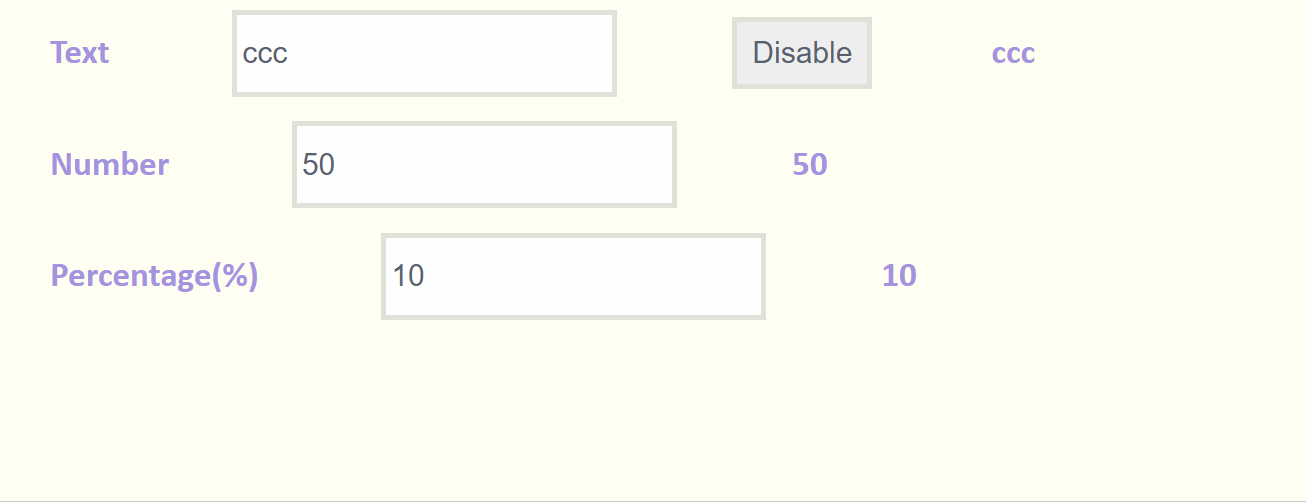﻿ Vue.js 带下拉选项的输入框(Textbox with Dropdown)组件_vue.js_脚本之家
vue.js# Vue.js 带下拉选项的输入框(Textbox with Dropdown)组件## 注册组件

```<script type="text/x-template" id="dropdown">
<div class="dropdown" v-if="options">
<!-- Dropdown Input -->
<input  :type="type"
:disabled="disabled"
v-model="input_value"
@focus="showOptions()"
@blur="exit()"
@keyup="keyMonitor"
@input="input_value = inputRule(type)" />
...
</script>
<script>
Vue.component('dropdown', {
template: '#dropdown',
props: {
type: String,
options: Array,
disabled: Boolean,
value: String
},
...
methods: {
inputRule:function(type){
var value;
switch(type){
case 'text':
value = this.input_value.replace(/[^a-zA-Z0-9]/g,'');
break;
case 'number':
value = this.input_value.replace(/^(?![+-]?\d+(\.\d+)?\$)/g,'');
break;
case 'percentage':
value = this.input_value.replace(/[^\d]/g,'');
value = value > 100 ? '100' : value;
value = value < 0 ? '0' : value;
break;
default:
console.log("no limitation");
}
return value;
},
...
</script>```

## 调用组件

```<dropdown   type = "text"
:options = "text_options"
:value = "text_value"
:disabled = "text_disabled"
@on_change_input_value = "onTextChange">
</dropdown>```

## 传递数据

• type: 输入框的类型，现支持 text， number 和 percentage。
• options： 输入框下拉列表的选项
• value: 输入框的值
• disabled: 是否禁止点击输入框

on_change_input_value： 更新值

```data: function () {
return {
text_value: 'ccc',
text_disabled: false,
text_options: [
{ id: 1, name: 'a' },
{ id: 2, name: 'bb' },
{ id: 3, name: 'ccc' },
{ id: 4, name: 'dddd' },
{ id: 5, name: 'eeeee' },
{ id: 6, name: 'fffff ' },
{ id: 7, name: 'gggggg' },
{ id: 8, name: 'hhhhhhh' },
{ id: 9, name: 'iiiiiiii' },
],
...
}
},
...
methods: {
onTextChange: function (new_text_value) {
this.text_value = new_text_value;
},
...
},```

GitHub